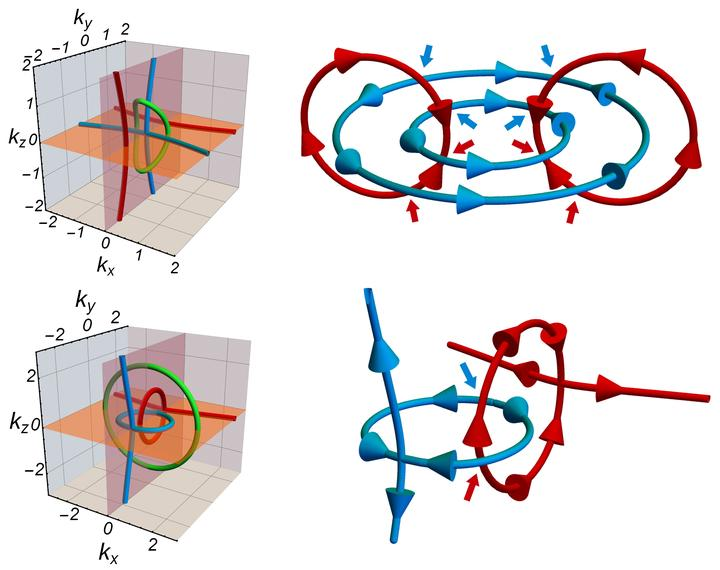Non-Abelian band topology in noninteracting metalsAbstract

Weyl points in three spatial dimensions are characterized by a Z-valued charge—the Chern number—which makes them stable against a wide range of perturbations. A set of Weyl points can mutually annihilate only if their net charge vanishes, a property we refer to as robustness. While nodal loops are usually not robust in this sense, it has recently been shown using homotopy arguments that in the centrosymmetric extension of the AI symmetry class they nevertheless develop a Z2 charge analogous to the Chern number. Nodal loops carrying a nontrivial value of this Z2 charge are robust, i.e., they can be gapped out only by a pairwise annihilation and not on their own. As this is an additional charge independent of the Berry π-phase flowing along the band degeneracy, such nodal loops are, in fact, doubly charged. In this manuscript, we generalize the homotopy discussion to the centrosymmetric extensions of all Atland-Zirnbauer classes. We develop a tailored mathematical framework dubbed the AZ+$\mathcal{I}$ classification and show that in three spatial dimensions such robust and multiply charged nodes appear in four of such centrosymmetric extensions, namely, AZ+$\mathcal{I}$ classes CI and AI lead to doubly charged nodal lines, while D and BDI support doubly charged nodal surfaces. We remark that no further crystalline symmetries apart from the spatial inversion are necessary for their stability. We provide a description of the corresponding topological charges, and develop simple tight-binding models of various semimetallic and superconducting phases that exhibit these nodes. We also indicate how the concept of robust and multiply charged nodes generalizes to other spatial dimensions.

Publication
Science 365, 1273—1277 (2019)